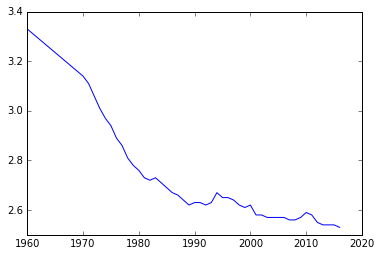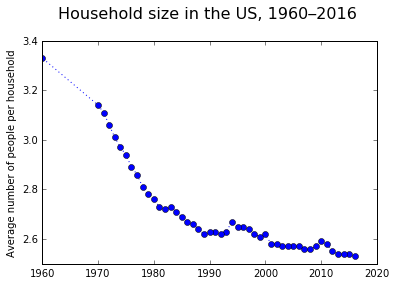# Python for Science¶

Python is free, it is open source, and it has a huge community.

Python is one of the most popular and loved programming languages in the world!

Many blogs come out every year listing the most popular programming languages. Python has been among the top choices for at least 5 years now. For example: The 7 Most In-Demand Programming Languages of 2018, by CodingDojo; or the post The Most In-Demand Programming Languages of 2018, on Third Republic.

Python can be used for many things: managing databases, creating graphical user interfaces, making websites, and much more… including science. Because of the many uses, the world of Python includes many, many Libraries (you load the parts that you need, when you need them).

In science, the two libraries that are king and queen of the world are: NumPy, and Matplotlib.

## Numpy¶

NumPy is for working with data in the form of arrays (vectors, matrices). It has a myriad built-in functions or methods that work on arrays directly. To load the library into your current session of interactive Python, into a saved Python script, or into a Jupyter notebook, you use:

import numpy


Tips:

• a one-dimensional array (vector) has the form: [1.0, 0.5, 2.5]
• a two-dimensional array (matrix) has the form: [[ 1.0, 0.5, 2.5], [ 0.5, 1.1, 2.0]]
• the elements in an array are numbered with an index that starts at 0
• the colon notation: in any index position, a : means "all elements in this dimension"
• once numpy is loaded, its built-in functions are called like this: numpy.function(arg) (where arg is the function argument: arrays to operats on, and parameters)

Try it!

In :
import numpy

In :
# By the way: comments in code cells start with a hash.
# here are two arrays, saved as variables x and y:
x = numpy.array([1.0, 0.5, 2.5])
y = numpy.array([[ 1.0, 0.5, 2.5], [ 0.5, 1.1, 2.0]])

In :
# The print function works on arrays:
print(x)

[ 1.   0.5  2.5]

In :
print(y)

[[ 1.   0.5  2.5]
[ 0.5  1.1  2. ]]

In :
numpy.shape(y)

Out:
(2, 3)
In :
numpy.shape(x)

Out:
(3,)

Let's review what happened there. We first loaded numpy, giving us the full power to use arrays. We created two arrays: x and y… then we print x and we print y. They look nice.

Numpy has a built-in function to find out the "shape" of an array, which means: how many elements does this array have in each dimension? We find that y is a two-by-three array (it has two dimensions).

What is the first element of x? We can use square brackets and the zero-index to find out:

In :
x

Out:
1.0

Exercise: Now, try it yourself. What is the first element of y?

In [ ]:



Right. The first element of y is a 3-wide array of numbers. If we want to access the first element of this now, we use:

In :
y

Out:
1.0

Exercise: Try picking out different elements of the array y

In [ ]:



### We learned that:¶

• The square brackets allow us to pick out the elements of an array using an index: x[i]
• For a two-dimensional array, we can use two indices: y[i][j]
• All indices start at zero.

This is super powerful!

## Matplotlib¶

Matplotlib is for making all kinds of plots. To get an idea of the great variety of plots possibe, have a look at the online Gallery. You can see that Matplotlib itself is a pretty big library. We can load a portion of the library (called a module) that has the basic plotting funtions with:

from matplotlib import pyplot


Once the pyplot module is loaded, its built-in functions are called like this: pyplot.function(arg) (where arg is the function argument).

## An example: size of households in the US¶

Did you know that the size of households—that is, the number of people living in each household—has been steadily decreasing in the US and many other countries? This has perhaps surprising consequences. Even if population growth slows down, or stops altogether, the number of households keeps increasing at a fast rate.

More households means more $CO_2$ emissions! This is bad for the planet.

### Get the data¶

Here, we're assuming that you have all the files from this tutorial, or are working on the lesson after launching Binder. In that case you have a dataset in the data folder.

To load the data into two arrays, named year and av-size, execute the following cell:

In :
#Load the data from local disk
year, av_size = numpy.loadtxt(fname='data/statistic_id183648.csv', delimiter=',',
skiprows=1, unpack=True)

In :
print(year)

[ 2016.  2015.  2014.  2013.  2012.  2011.  2010.  2009.  2008.  2007.
2006.  2005.  2004.  2003.  2002.  2001.  2000.  1999.  1998.  1997.
1996.  1995.  1994.  1993.  1992.  1991.  1990.  1989.  1988.  1987.
1986.  1985.  1984.  1983.  1982.  1981.  1980.  1979.  1978.  1977.
1976.  1975.  1974.  1973.  1972.  1971.  1970.  1960.]


Exercise: Now print the variable av_size, correspondig to the average size of households (in numbers of people) for each year:

In [ ]:



Great! The next thing we want to do is make a plot of the changing size of households over the years. To do that, we need to load the Matplotlib module called pyplot:

In :
from matplotlib import pyplot
%matplotlib inline


What's this from business about? matplotlib is a pretty big (and awesome!) library. All that we need is a subset of the library for creating 2D plots, so we ask for the pyplot module of the matplotlib library.

Plotting the data is as easy as calling the function plot() from the module pyplot.

In :
pyplot.plot(year, av_size)

Out:
[<matplotlib.lines.Line2D at 0x10ff57978>]But what if we'd like to get a title on this plot, or add labels to the axes? (We should always have labelled axes!). Also, we notice a long jump from the year 1960 to 1970: let's add markers to the plot and change the line style to a dotted line.

In :
pyplot.plot(year, av_size, linestyle=':', marker='o')
pyplot.title("Household size in the US, 1960–2016 \n", fontsize=16)
pyplot.ylabel("Average number of people per household")

Out:
<matplotlib.text.Text at 0x110238fd0>Exercise: In the same cell above, now add a label on the x-axis, using the pyplot.xlabel() function, and re-execute it.

In [ ]:



## Python for science, so far¶

You learned about:

• loading the Python libraries for science
• using data in the form of arrays with the numpy library
• accessing elements of an array
• loading data from a file
• plotting data with the Matplotlib library
• adding title, labels to a plot, and changing the style

# Next¶

(c) 2017 Lorena A. Barba, Natalia Clementi. Free to use under the Creative Commons Attribution CC-BY 4.0 License. Written for the tutorial "Data Science for a Better World", at the GW _Caminos al Futuro_ Summer program.